# 1. Simplify, if possible. Write your answer as a power or as a product of powers

 g.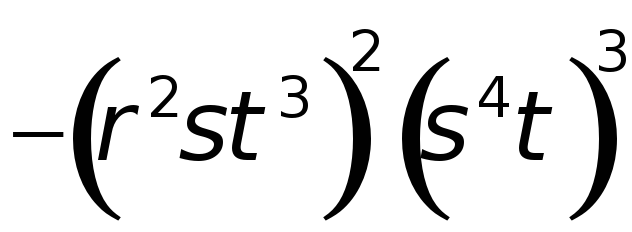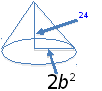2. The volume of a cone is given by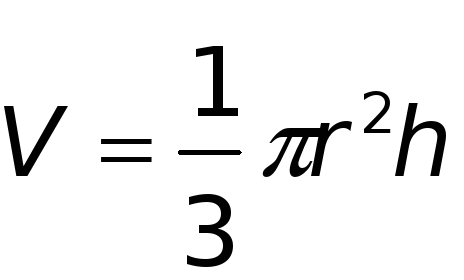where r is the radius of the base, h is the height, and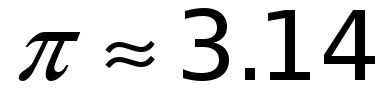. What is the volume of the cone (on the right) in terms of b? 3. The power generated by a windmill can be modeled by the equation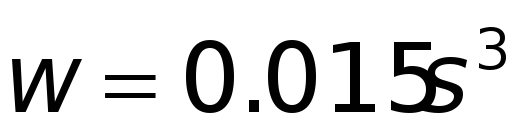, where w is the power measured in watts and s is the wind speed in miles per hour. a. Find the ratio of the power generated by a windmill when the wind speed is 20 miles per hour to the power generated when the wind speed is 10 miles per hour.Download 1.03 Mb.Share with your friends: